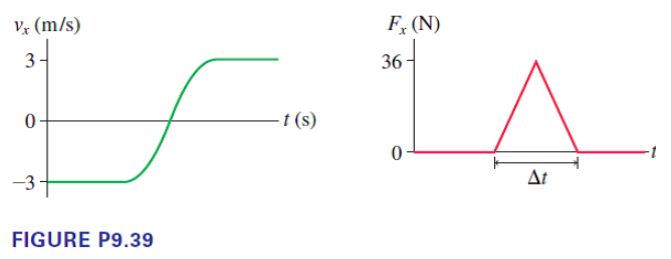# Problem: A 600 g air-track glider collides with a spring at one end of the track. figure P9.39 shows the glider's velocity and the force exerted on the glider by the spring. How long is the glider in contact with the spring?

###### FREE Expert Solution

Impulse due to applied force, Fx is given by:

$\overline{){\mathbf{J}}{\mathbf{=}}\frac{\mathbf{1}}{\mathbf{2}}{{\mathbf{F}}}_{{\mathbf{x}}}{\mathbf{∆}}{\mathbf{t}}}$

Impulse on an object:

$\overline{){\mathbf{J}}{\mathbf{=}}{\mathbf{m}}{\mathbf{\left(}}{{\mathbf{v}}}_{{\mathbf{f}}}{\mathbf{-}}{{\mathbf{v}}}_{{\mathbf{o}}}{\mathbf{\right)}}}$

Equating:

(1/2)FxΔt = m(vf - v0)

Δt = 2m(vf - v0)/Fx

93% (302 ratings)###### Problem Details

A 600 g air-track glider collides with a spring at one end of the track. figure P9.39 shows the glider's velocity and the force exerted on the glider by the spring. How long is the glider in contact with the spring?